# Paper ICT2014 .pdf

### File information

Original filename: Paper ICT2014.pdf
Title: Microsoft Word - Paper ICT2014.docx

This PDF 1.3 document has been generated by Word / Mac OS X 10.9.1 Quartz PDFContext, and has been sent on pdf-archive.com on 23/09/2014 at 20:25, from IP address 83.57.x.x. The current document download page has been viewed 525 times.
File size: 1.3 MB (5 pages).
Privacy: public file

Paper ICT2014.pdf (PDF, 1.3 MB)

### Document preview

On the Design of Spreading Sequences for CDMA
Systems with Nonlinear OQPSK-type Modulations
Ângelo da Luz (1,2), Francisco Cercas (1,2), Pedro Sebastião (1,2) and Rui Dinis (2,3)
(1)

ISCTE-IUL, Lisbon University Institute, Portugal
IT, Instituto de Telecomunicações, Portugal
(3)
FCT-UNL, Monte da Caparica, Portugal

(2)

Abstract—The main focus of this paper is to present an analytical
method for calculate the correlation between OQPSK (Offset
Quadrature Phase-Shift Keying) signals modulated by several
spreading sequence families. Our analytical model includes
nonlinearities, namely by treating the transmitter amplifier as
highly nonlinear. Grossly nonlinear power amplification is highly
desirable as it allows power-efficient low-cost receivers, with
applications in satellite, underwater or deep space
communications. OQPSK-type modulations include as special
cases CPM (Continuous Phase Modulation) schemes and can be
designed to have low envelope fluctuations or even a constant
envelope, essential in many communications systems (e.g.,
satellite). This model and the derived analytical results for the
correlation of sequences in such a nonlinear environment are
then applied to a DS-CDMA (Direct Sequence – Code Division
Multiple Access) system. We present simulation results for three
types of spreading sequences: ML (Maximum-Length), Kasami
and TCH (Tomlinson, Cercas, Hughes) sequences.
The
simulation results are presented to compare the performance of
those types of sequences in the linear and nonlinear DS-CDMA
systems.
Keywords – Nonlinear systems, spreading sequences, powerefficient communications, correlation

I. INTRODUCTION
There are many systems that are inherently limited by the
power source, so their efficiency must be maximized,
although there is a current trend towards generalized green
communications. The most critical ones, such as satellite,
underwater, deep space communications or simply mobile
terminals, should not only use efficient modulations, but also
grossly nonlinear amplifiers, as these can help save a huge
amount of power.
Since most results known until recently tend to consider
systems as linear as possible, namely the transmitter amplifier,
the correlation of spreading sequences after modulation in a
nonlinear system is not known, although nonlinear distortions
caused by the amplifier are sometimes considered, although
isolated from the other mentioned factors. Furthermore, it is
possible to improve the spectral efficiency and to reduce the

impact of nonlinearities by considering others types of
(Phase-Shift Keying).
As referred, an obvious nonlinear component of the
transmitting chain is the amplifier. In this study, our model
assumes it as definitely nonlinear. In fact, the use of nonlinear
amplifiers reduces the complexity and cost of systems and it
also increases their efficiency and autonomy, as for example
on mobile terminals which depend on batteries. These
amplifiers are less complex, simpler to implement and have
higher amplification efficiency and output power than linear
amplifiers, which is important in most communications
systems, such as wireless communications. However, nonconstant envelope signals should not be amplified by grossly
nonlinear amplifiers in order to avoid nonlinear distortion
effects. CPM (Continuous Phase Modulation) schemes  are
constant or almost-constant envelope modulations that include
as special cases MSK (Minimum Shift Keying)  and
GMSK (Gaussian MSK)  modulations, among others.
These modulations provide high power and spectral efficiency.
They are OQPSK-type modulations as they can be
decomposed as the sum of OQPSK components [4, 5] keeping
their OQPSK-type structure when submitted to band pass
memory-less nonlinear devices.
Pseudorandom sequences are widely used in many
communications systems, e.g., satellite systems, whose
performance is dictated by their correlation behavior. For
example, to keep cross-correlation as small as possible in most
system applications, many families of pseudorandom
sequences have been investigated, so as to improve the
performance of the communication system under study.
However, the majority of these studies usually assume ideal
conditions that can be easily modeled and studied, such as
linear modulations and ideal linear transmitters (i.e., with
linear amplifiers), which do not exist in the real world. For
this purpose, we have analytically derived the real correlation
of several types of sequences after being processed by a
nonlinear system. We then extend and apply these expressions
to a DS-CDMA (Direct Sequence – Code Division Multiple

Access) model and finally we use Monte Carlo method to
simulate the system for both linear and nonlinear conditions,
comparing the performance of ML (Maximum-length) ,
Kasami  and TCH (Tomlinson, Cercas, Hughes) 
sequences and evaluating their BER (Bit Error Rate)
performance in a CDMA nonlinear system, as previously
described.
II. PARALLEL AND SERIAL OQPSK SCHEMES
OQPSK-type modulations can be represented in both
serial and parallel formats. These types of modulations are
derived from QPSK (Quaternary Phase-Shift Keying) where

xs (t ) = x p (t )e

⎡⎛
⎤ − jπ t
⎞
xs (t ) = ⎢⎜ ∑ anpδ (t − nT ) ⎟ * rP (t ) ⎥ e 2T
⎠
⎣⎝ n
⎦

t
t
− jπ
⎡⎛
⎤
⎞ − jπ ⎤ ⎡
xs (t ) = ⎢⎜ ∑ anpδ (t − nT ) ⎟ e 2T ⎥ * ⎢rp (t )e 2T ⎥ =
⎠
⎣⎝ n
⎦ ⎣
⎦
p
n

= ∑a e

carrier frequency and x p (t ) the complex envelope given by

(6)

− jπ

t
2T

rp (t − nT )e

− jπ

t
2T

(7)

n

(1)

n

where

(5)

The order of the translation and convolution operations can be
changed enabling the following mathematical deduction

}

x p (t ) = ∑ an rp (t − 2nT )

t
2T

substituting x p (t ) according to (3), gives

the band pass signal is xBP (t ) = Re x p (t )e 2 jπ fc t , f c is the

{

− jπ

The complex envelope of OQPSK signal in the serial format
can then be written as

an = anI + janQ with anI = ±1 and anQ = ±1 represent

xs (t ) = ∑ ans rs (t − 2nT )

the ‘in-phase’ and ‘quadrature’ bits and rp (t ) is the adopted

(8)

n

pulse shape, where T is the bit duration. QPSK symbols are
and, using equation (7), we can conclude that
separated by 2T because we are transmitting 2 bits per symbol.
Let us now consider the transmission of the same band
t
− jπ
2T
(9)
r
(
t
)
=
r
(
t
)
e
,
pass signal but now assuming OQPSK-type schemes. This
s
p
modulation is also based on the transmission of two pulses of
n
− jπ
2T time duration but with a delay T between them. The
(10)
ans = anp e 2
complex envelope of the signal represented in parallel format
is given by 
which means that, ans always presents a real value ans = ±1,

x p (t ) = ∑ anI rp (t − 2nT ) + ∑ anQ rp (t − 2nT − T )
n

(2)

n

A compact way to represent the same signal, is given by

x p (t ) = ∑ anp rp (t − nT )

(3)

n

enabling the use of sequences with only real values because
only a real sequence of symbol coefficients is needed, rather
than sequences with real and imaginary values, which are
used in the parallel format. This serial format also allows both
the serial modulator and demodulator to have a single-branch
structure .

where anp corresponds alternately to the “in-phase” and

III. NONLINEAR OQPSK MODULATIONS

I
n/2

⎧a = ±1
⎪
a = ⎨
⎪ ja Q
⎩ ( n +1)/ 2 = ± j

, n even
(4)

p
n

, n odd

To obtain the serial format, we consider a carrier frequency
fc ' = fc + 1/ 4T . In this case the band pass signal is

{

'

}

xBP (t ) = Re xs (t )e2 jπ fc t where

Let us now consider the amplification of OQPSK signals
over nonlinear band pass systems, using a nonlinear band pass
memoryless amplifier. The signal at the amplifier input is
expressed by

xin (t ) = Re j arg( xin (t ))
where

(11)

R =| xin (t ) | is the envelope. At the output, the complex

envelope is given by 

xout (t ) = A(R)e j (Θ( R)+arg( xin (t )))

(12)

where  𝐴 𝑅 and 𝛩 𝑅  denote the AM-to-AM and AM-to-PM
conversion functions, respectively, of the nonlinear amplifier.
We modeled the amplifier as an ideal BPHL (Band-Pass Hard
Limiter) where 𝐴 𝑅 = 1 and  Θ 𝑅 = 0, which is the worst
case scenario for nonlinear distortion. Thus, the complex
envelope at the output of the nonlinear amplifier can be
simplified to

xout (t ) = e j (arg( xin (t )))

(13)

Considering now the following signal at the input of the
nonlinear device

xin (t ) = ∑ an r (t − nT )

(14)

IV. SIMULATION MODEL
In this paper we consider the downlink transmission in a
DS-CDMA system in which a base station (BS)
simultaneously transmits data blocks for P users. Each bit of
the data blocks, will be spread considering three types of
signal, employing the same spreading sequences, to compare
their performance.
To simulate the system, we assume a simple model for the
transmitter of a BS, where data is spread considering ML,
Kasami and TCH sequences, modulated by MSK and GMSK,
followed by a nonlinear amplifier as shown in Figure 2.

n

where r (t ) is the pulse of the used modulation. Since we are
assuming a serial representation of the OQPSK signal, the an
values only take real values, that is, an = ± 1.
If the OQPSK signal does not have constant envelope,
extra-components will appear after nonlinear amplification,
but interestingly, the resulting nonlinear signal can be
decomposed as the sum of OQPSK components. Thus, the
signal at the output of the nonlinear device is given by 
M −1

xout (t ) = ∑ ∑ an( m ) r ( m ) (t − nT )

(15)

m=0 n

Figure 2 - Block diagram for the DS-CDMA transmitter.

For a GMSK pulse, the transmitted signal at the output of
the BS is given by
P −1 M −1

xNL (t ) = ∑∑∑ b p an( m, p ) r ( m, p ) (t − nT )

(16)

p =0 m =0 n

where the number of components after
quantified by variable M . In this study
the four most important components, i.e.,
shows variables r (0) (t ) , r (1) (t ) , r (2) (t ) and
with the resulting components
amplification of the pulse r (t ) .

from

amplification, is
we only consider
M = 4 . Figure 1,
r (3) (t ) associated
the

nonlinear

where b is the information bit of user p .
To recover the transmitted bits of user p we must correlate
all other users, nonlinear effects and noise, with the same
sequence used at the transmitter. We assume an AWGN
(Additive White Gaussian Noise) channel represented by n(t ) .
We consider the receiver structure shown in Figure 3.

Figure 3 – Block diagram for the receiver.

Figure 1 - Pulse shapes

,

,

,

and

.

Since the main operation of the receiver is the crosscorrelation of sequences in this nonlinear environment, we
now present an analytical method to evaluate the correlation
of sequences using nonlinear OQPSK-type modulations,

applied to DS-CDMA systems. This allows us to compare and
to determine the behavior and characteristics of pseudorandom sequences in this non-linear multi-user system.

If the pulse type used, e.g., a MSK pulse, complies with
Nyquist conditions, i.e., p(kT ) = 0, k ≠ 0 , then this correlation
is given by

Let us now consider the DS-CDMA signal at the output of
the transmitter, shown in Figure 2

xNL (t ) =

P −1 M −1

∑ ∑ ∑b

p'

( m ', p ') ( m ', p ')
n'

a

P -1

SF −1

p' = 0

n =0

Rx (τ ) = ∑ bp ' ∑ an ', p ' an*, p

B. Correlation of binary sequences

r

(t − nT )

(17)

p '=0 m '=0 n '

and the nonlinear signal from user p

V. PERFORMANCE RESULTS
In this section we present the performance results for each
pseudorandom sequence type together with the effects of
nonlinearity, by comparing the obtained results for linear and
non-linear systems. Figure 4 presents the autocorrelation of
each sequence after nonlinear modulation and amplification,
evaluated according to our analytical expressions.
MLS

M −1

x(t ) = ∑ ∑ an( m, p ) r ( m, p ) (t − nT )

(18)

Rx (τ ) = ∫

NT

0

xNL (t ) x* (t − τ )dt

(19)

clearly,

P -1
⎛ M −1 M −1
⎞
Rx (τ ) = ∑ bp' ⎜ ∑ ∑ ∑ Rmm'p ' (n) pmm'p ' (t − (n '− n)T − (τ p ' − τ p )) ⎟
(20) ⎠
p'
⎝ m = 0 m' = 0 n

Magnitude (normalized)

m=0 n

the correlation between these signals is given by

(24)

KASAMI

TCH

1

1

1

0.8

0.8

0.8

0.6

0.6

0.6

0.4

0.4

0.4

0.2

0.2

0.2

0

0

0

-0.2
-n/2 -n/4 0 n/4 n/2
Code Delay (samples)

-0.2
-n/2-n/4 0 n/4 n/2
Code Delay (samples)

-0.2
-n/2-n/4 0 n/4 n/2
Code Delay (samples)

Figure 4 - Autocorrelation function of all OQPSK components for each
pseudorandom sequence.

where
N −1

Rmm'p ' (n) = ∑ an( m''− n, p ') an'( m, p )*

(21)

pmm'p ' (t ) = r ( m' , p ') (−t )r ( m , p )* (t )

(22)

n' = 0

and

As we can see, TCH sequences present the best
performance on its autocorrelation in this nonlinear scenario.
In order to evaluate the performance of ML, Kasami and TCH
sequences in a DS-CDMA system, for both linear and
nonlinear conditions, we used Monte Carlo simulation.

In our model we assume that the spreading sequences for
the interfering users are randomly delayed, so an, p ' = an −ΔN , p
where ΔN represents a random variable uniformly distributed
between zero and the length of the employed spreading
sequences. We then conclude that,

Rx (τ ) =

P −1

⎛ M −1 M −1

∑ b ⎜⎝ ∑ ∑ ∑ R
p'

p ' =0

mm'p '

m = 0 m' = 0 n

⎞
(n) pmm'p ' (t − (n '− n)T ) ⎟
⎠

(23)

Figure 5- Cumulative distribution of the received bits in linear and
NL conditions.

Figure 5 presents the cumulative distribution of the received
bits for both systems, using the same parameters. As expected,
in the nonlinear system the dispersion is higher and the
majority of the received bits in the linear system are closer to
the right decision value. Figure 6 shows and compares the
results obtained for ML, Kasami and TCH sequences in both
systems.

VI. CONCLUSIONS
In this paper we presented the performance of ML, TCH
and Kasami sequences for a system modeled as the downlink
of a DS-CDMA system using OQPSK-type modulations and
highly nonlinear amplification, in a AWGN channel. The
model used is based on the analytical evaluation of correlation
for these nonlinear systems, which was derived, followed by
Monte Carlo simulation, to evaluate the system’s performance
and capacity at BER=10-3. Both linear and nonlinear systems
were simulated. The results have shown that TCH sequences
present better performance on both systems, allowing a
capacity of 25 simultaneous users in those highly nonlinear
conditions.
ACKNOWLEDGEMENTS
This work was partially supported by FCT/ Instituto de
Telecomunicações projects OE/EEI/LA0008/2011 and
PTDC/EEA-TEL/120666/2010.
REFERENCES








Figure 6 - Performance of sequences in a DS-CDMA system.

In this study we considered sequences length 256, that is, a
spreading factor of SF = 256 , for which we tried to maximize
the system’s capacity. We also assumed a value for the BER
of BER = 10-3 , which is usually taken as the minimum value
for the QoS (Quality Of Service) in many applications. As
previously mentioned, and to get a more realistic scenario, we
considered random delays for interfering users. We found that
TCH sequences allow the maximum capacity in both linear
and nonlinear systems, which is about 25 simultaneous users.
It is clear that MAI (Multiple Access Interference) and
nonlinearities degrade the system’s performance as we should
expect for all types of sequences. ML sequences have the
worst behavior, which is due to their bad cross-correlation
properties. On the other hand, we can see that TCH sequences
outperform the other sequence types considered. This
behavior is consistent for both linear and nonlinear situations.










T. Aulin, "Continuous phase modulation - Part II: partial response
signaling," in IEEE Transactions on Communications, vol. 29, no. 3,
1981, pp. 210-225.
S. Gronemeyer and A. McBride, "MSK and offset QPSK modulation,"
in IEEE Transactions on Communications, vol. 24, no. 8, 1976, pp. 809820.
telephony," in IEEE Transactions on Communications, vol. 29, no. 7,
1981, pp. 1044-1050.
P.A. Laurent, "Exact and approximate construction of digital phase
modulations by superposition of amplitude modulated pulses (AMP),"
in IEEE Transactions on Communications 1986; 34(2): pp. 234–237.
A. Gusmao, V. Gonçalves and N. Esteves, "A novel approach to
modeling of OQPSK-type digital transmission over nonlinear radio
channels," IEEE Journal on Selected Areas in Communications, vol. 15,
no. 4, 2002, pp. 647-655.
A. J. Viterbi, CDMA: Principles of Spread Spectrum Communication.
Electricity and Magnetism, 3rd ed., vol. 2. Oxford: Clarendon, 1892,
pp.68–73.
F. Cercas, M. Tomlinson and A. Albuquerque, “TCH: A New Family of
Cyclic Codes Length 2m”, Proc. IEEE International Symposium on
Information Theory, Hilton Palacio del Rio Hotel, San Antonio, Texas,
USA, pp. 198, 17-22 January, 1993.
J.G. Proakis and Masoud Salehi, Digital communications, 5th edition,
McGraw- Hill, 2007.
Palhau A, Dinis R, Gusmão A. Modelling and Evaluation of DS/SSMA
Systems Employing Nonlinearly Distorted MSK-type Modulated
Signals, In 4th International Conference on Conceptual Structures ICCS
’96, Singapore, 1996.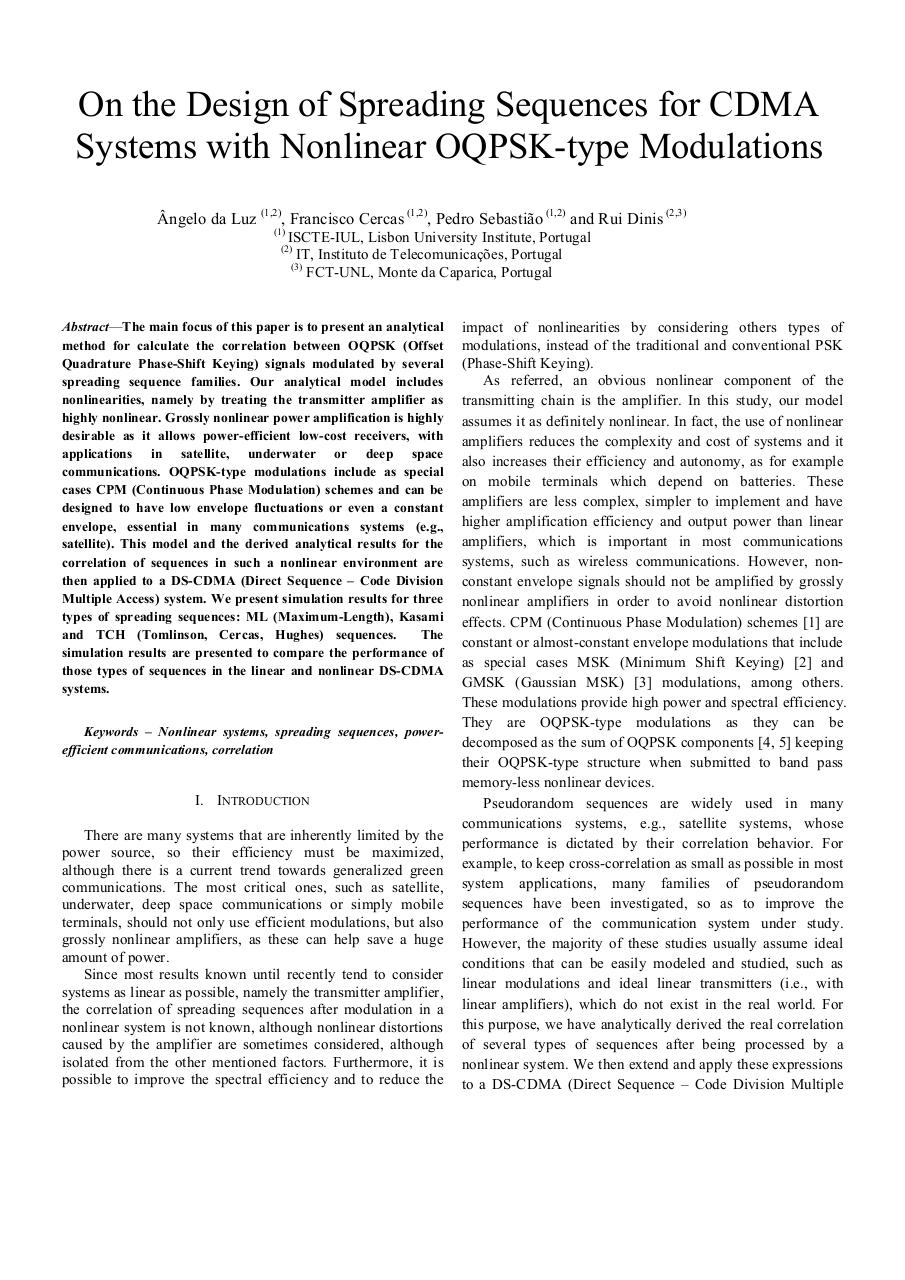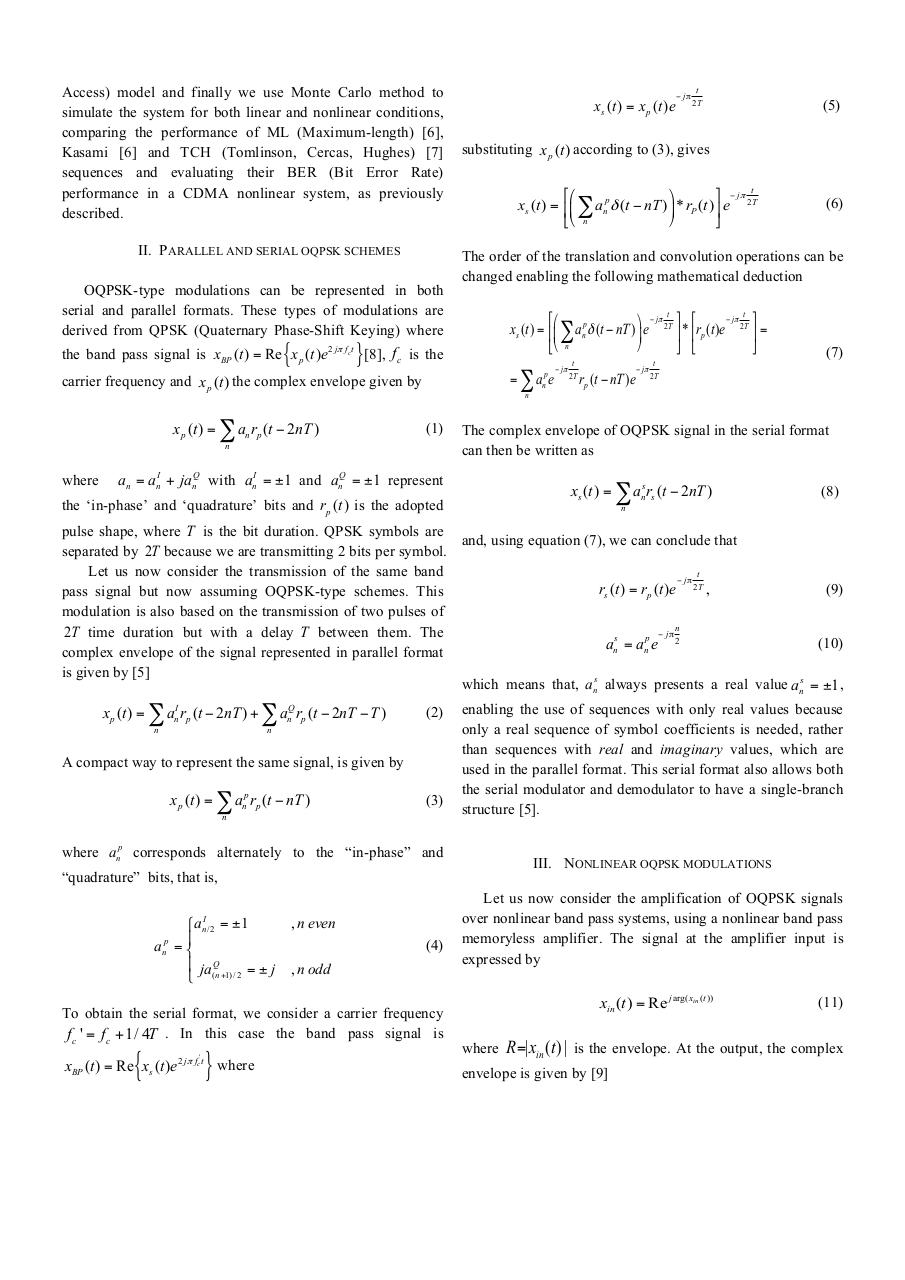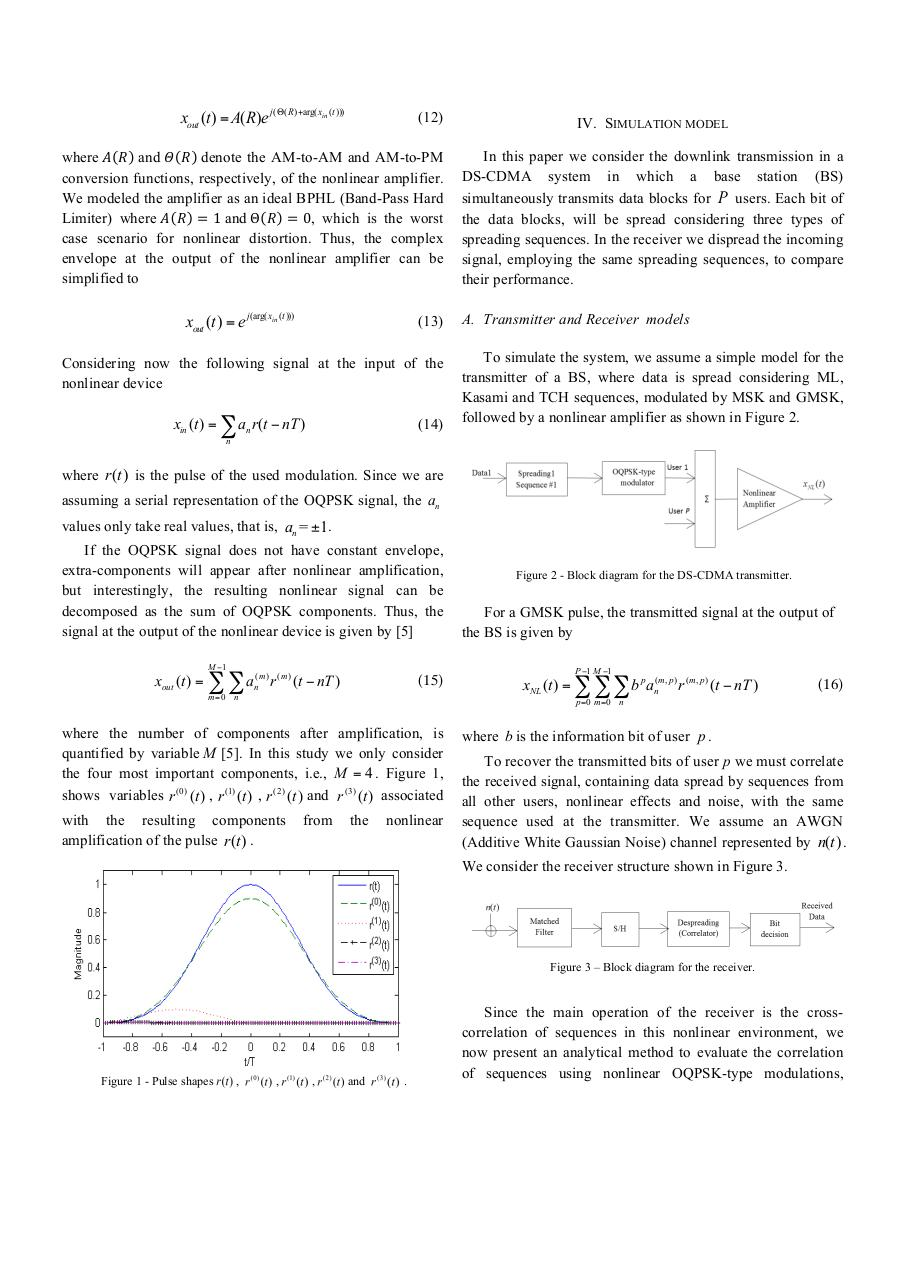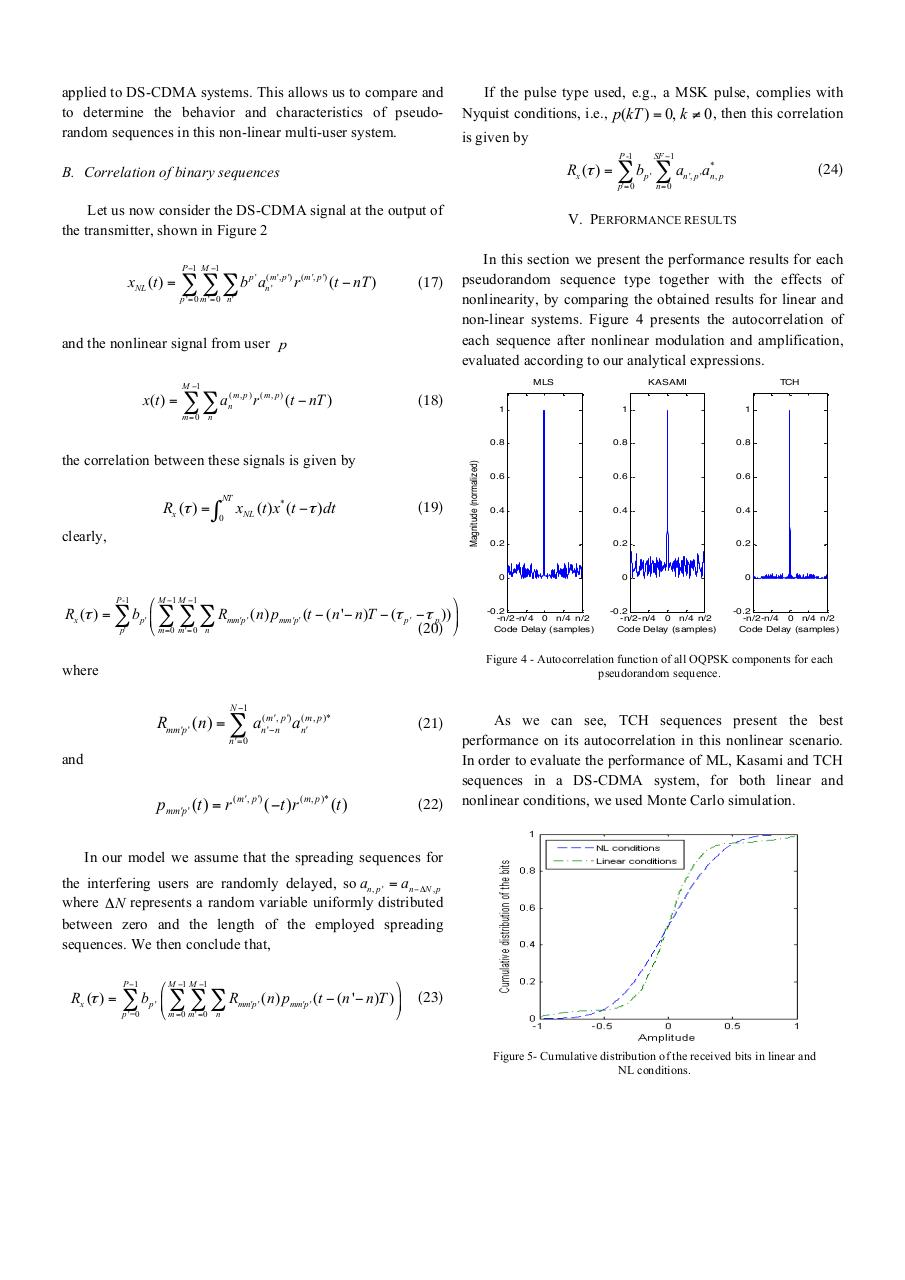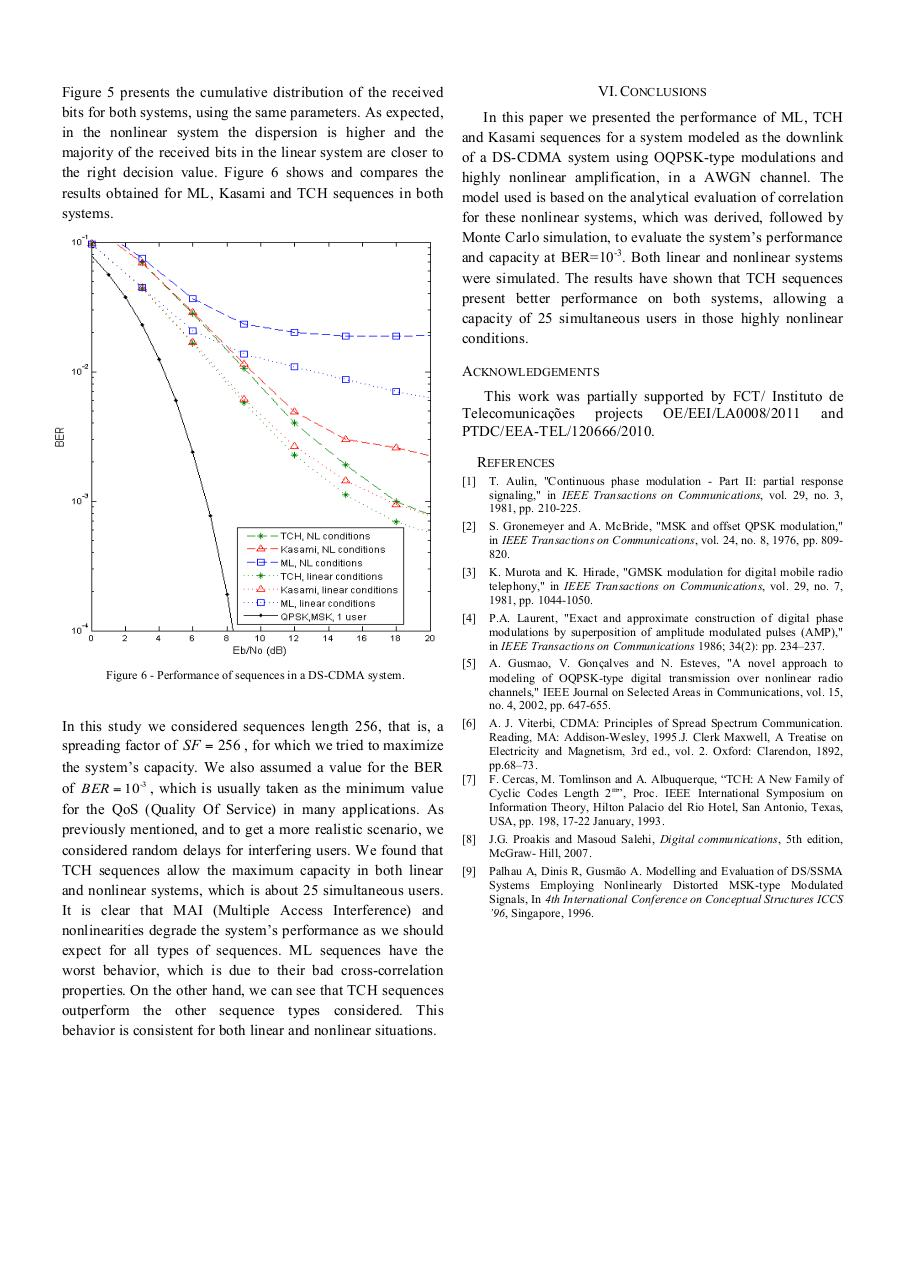#### HTML Code

Copy the following HTML code to share your document on a Website or Blog

#### QR Code### Related keywords